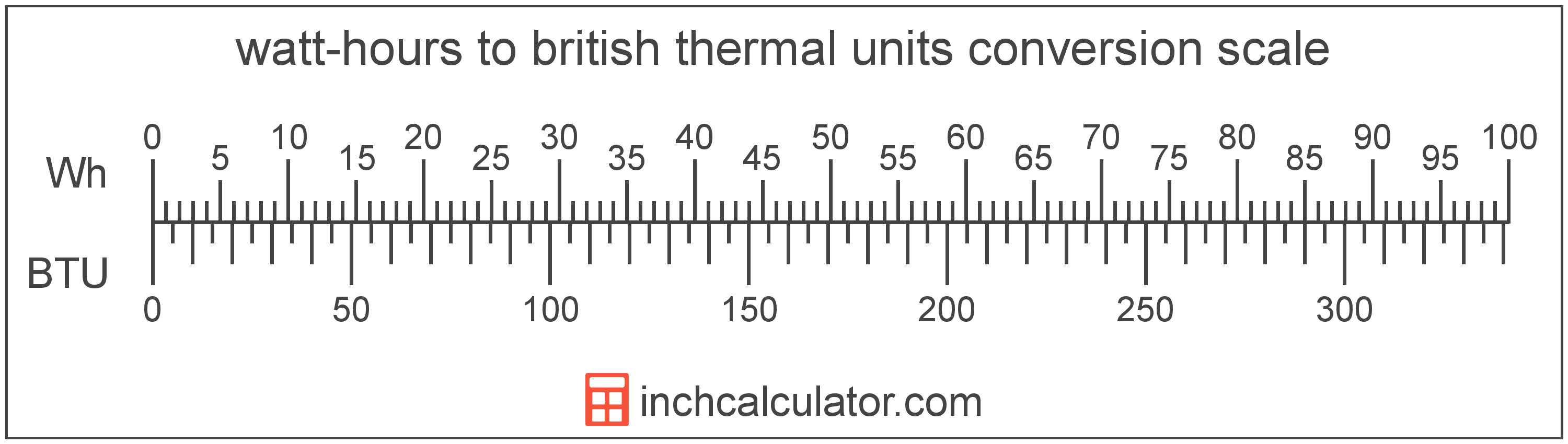# Watt-hours to British Thermal Units Conversion

Enter the energy in watt-hours below to get the value converted to british thermal units.

Results in British Thermal Units:1 Wh = 3.412142 BTU

## How to Convert Watt-hours to British Thermal UnitsTo convert a watt-hour measurement to a british thermal unit measurement, multiply the energy by the conversion ratio. One watt-hour is equal to 3.412142 british thermal units, so use this simple formula to convert:

british thermal units = watt-hours × 3.412142

The energy in british thermal units is equal to the watt-hours multiplied by 3.412142.

For example, here's how to convert 5 watt-hours to british thermal units using the formula above.
5 Wh = (5 × 3.412142) = 17.060708 BTU

## Watt-hours

The watt-hour is a measure of electrical energy equal to one watt of power over a one hour period.

Watt-hours can be abbreviated as Wh, for example 1 watt-hour can be written as 1 Wh.

## British Thermal Units

British thermal units are a measure of heat energy. Specifically, one british thermal unit is equal to the amount of heat energy required to increase the temperature of one pound of water one degree Fahrenheit. They are often used as a way to rate the energy heat producing appliances such furnaces, and to price heating fuels such as natural gas.

The british thermal unit is a US customary unit of energy. British thermal units can be abbreviated as BTU, for example 1 british thermal unit can be written as 1 BTU.

## Watt-hour to British Thermal Unit Conversion Table

Watt-hour measurements converted to british thermal units
Watt-hours British Thermal Units
1 Wh 3.4121 BTU
2 Wh 6.8243 BTU
3 Wh 10.24 BTU
4 Wh 13.65 BTU
5 Wh 17.06 BTU
6 Wh 20.47 BTU
7 Wh 23.88 BTU
8 Wh 27.3 BTU
9 Wh 30.71 BTU
10 Wh 34.12 BTU
11 Wh 37.53 BTU
12 Wh 40.95 BTU
13 Wh 44.36 BTU
14 Wh 47.77 BTU
15 Wh 51.18 BTU
16 Wh 54.59 BTU
17 Wh 58.01 BTU
18 Wh 61.42 BTU
19 Wh 64.83 BTU
20 Wh 68.24 BTU
21 Wh 71.65 BTU
22 Wh 75.07 BTU
23 Wh 78.48 BTU
24 Wh 81.89 BTU
25 Wh 85.3 BTU
26 Wh 88.72 BTU
27 Wh 92.13 BTU
28 Wh 95.54 BTU
29 Wh 98.95 BTU
30 Wh 102.36 BTU
31 Wh 105.78 BTU
32 Wh 109.19 BTU
33 Wh 112.6 BTU
34 Wh 116.01 BTU
35 Wh 119.42 BTU
36 Wh 122.84 BTU
37 Wh 126.25 BTU
38 Wh 129.66 BTU
39 Wh 133.07 BTU
40 Wh 136.49 BTU